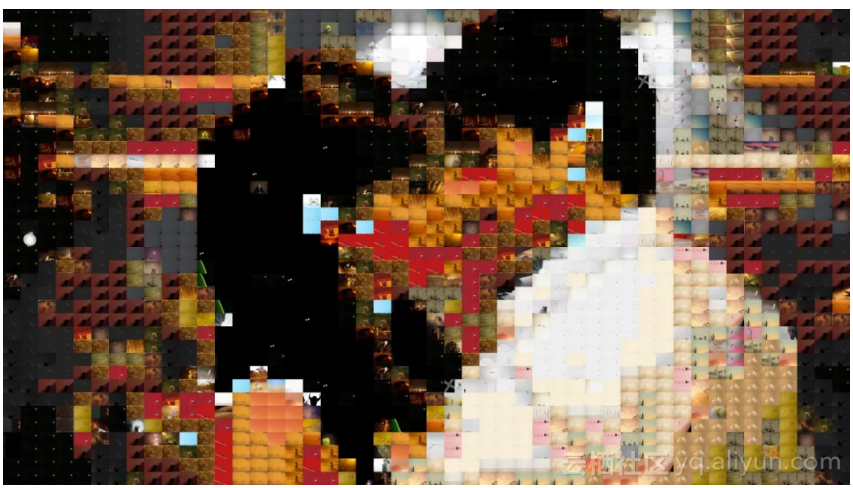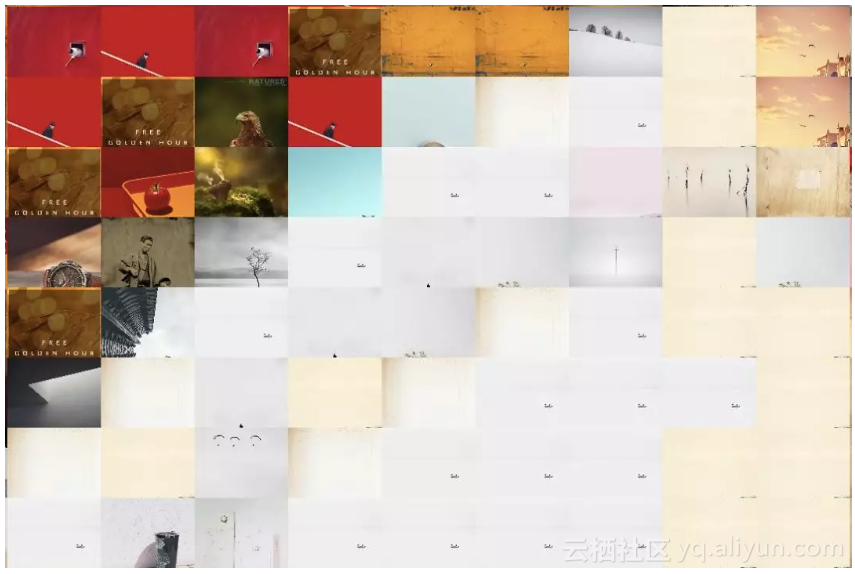用Python生成马赛克画

+关注继续查看import re
import os
import cv2
import numpy as np
from tqdm import tqdm

IMG_DIR = "images"

img_dir = IMG_DIR
filenames = os.listdir(img_dir)
result = []
print(len(filenames))
for filename in tqdm(filenames):
if not re.search(".jpg", filename, re.I):
continue
try:
filepath = os.path.join(img_dir, filename)
row = im.shape
col = im.shape
im = resize(im, tile_row, tile_col)
result.append(np.array(im))
except Exception as e:
msg = "error with {} - {}".format(filepath, str(e))
print(msg)
return np.array(result, dtype=np.uint8)

tile_row, tile_col = get_tile_row_col(img.shape)
for row in range(0, img_shape, tile_row):
for col in range(0, img_shape, tile_col):
roi = img[row:row+tile_row,col:col+tile_col,:]

from scipy.spatial.distance import euclidean
def img_distance(im1, im2):
if im1.shape != im2.shape:
msg = "shapes are different {} {}".format(im1.shape, im2.shape)
raise Exception(msg)
array1 = im1.flatten()
array2 = im2.flatten()
dist = euclidean(array1, array2)
return dist

im1和im2是两张图片的数据，图片数据是一个三维的numpy数组，这里我们将三维数组转换成一维数组后，比较两者的欧式距离。之后要找出最相似的图片，只需遍历图片集中所有的图片，找到距离最短的那张图片，去替换原图中的小方格就可以了。23 08472 0979 010273 0python 迭代器和生成器
920 0Python生成一个不含回文字符串的字符串
[本文出自天外归云的博客园] 回文字符串介绍 回文字符串就是对称的字符串，例如： “ABA” “ABBA” “ABCBA” 题目 给定一个字符串，请发明一种方法，让字符串中不包含回文字符串。
852 0python生成和解析二维码
1127 011388 0752 0python 列表生成器
1660 0

7208

9

+ 订阅

《2021云上架构与运维峰会演讲合集》

《零基础CSS入门教程》

《零基础HTML入门教程》